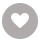# 江山如画电子书

《江山如画》诗集，是一部追溯历史评古论今的名篇佳作。

2人正在读 | 0人评论6.4

• 读书简介
• 目录
• 累计评论(70条)
• 读书简介
• 目录
• 累计评论(70条)

（威虎山）

（林海雪原）

（鸡冠山）

（凤凰山）

（凤凰山四季景）

（二龙山）

（香炉山）

（香炉山）

（呼白山）

（锅盔山）

（毛公山）

（毛公山）

（大兴安岭）

（完达山）

（老秃顶子）

（套环山）

（净月潭）

（向海湿地）

（帽儿山）

（长白山）

（天池）

（长白山）

（天鼎山）

（东北虎园）

（防川村）

（世界雕塑园）

（三角龙湾）

（拉法山）

（五女峰）

（掉水壶瀑布）

（掉水湖瀑布）

（医巫闾山）

（千山）

（凤凰山）

（老秃顶子）

（天华山）

（朝阳凤凰山）

（海棠山）

（铁刹山）

（关门山）

（望儿山）

（阿尔山）

（苏木山）

（苏木山）

（喇嘛山）

（乌拉山）

（贺兰山）

（贺兰山）

（赤峰红山）

（卓资山）

（大兴安岭）

（阴山）

（岩画）

（大青山）

（九峰山）

（草原）

（祖山）

（雾灵山）

（狼牙山）

（金山岭长城）

（小五台山）

（野三坡）

（白石山）

（天柱峰）

（苍岩山）

（驼梁山）

（灵山）

（海坨山）

（百花山）

（八达岭长城）

（香山）

（百望山）

（红螺山）

（云蒙山）

（妙峰山）

（鹫峰）

（盘山）

（盘山大佛）

（梨木台景区）

（黄崖关长城）

（九顶山）

（九龙山）

（白蛇谷）

（神与凡）

（八仙山）

（翠屏山）

（黄花山）

（府君山）

（石龙峡）

（泰山）

（泰山十八盘）

（泰山）

（登岳）

（泰山观日）

（泰山十八盘）

（沂蒙山）

（崂山）

（龙口南山）

（原山）

（莲花山）

（莲山•赋）

（千佛山）

（佛）

（仙姑顶）

（鲁山）

（峄山）

（齐山）

（博山）

（嵩山）

（嵩山览胜）

（少林武术）

（王屋山）

（愚公移山）

（老君山）

（鸡公山）

（白云山）

（云台山）

（尧山）

（恋人石）

（云梦山）

（万仙山）

（嵖岈山）

（穹窿山）

（虎丘山）

（虎丘山）

（天平山）

（天池山）

（缥缈峰）

（灵岩山）

（凤凰山）

（石公山）

（紫金山）

（花果山）

（花果山）

（水帘洞）

（神与凡）

（黄山）

（黄山）

（迎客松）

（迎客松）

（傲骨石）

（妙笔生花）

（迎客松）

（黄山颂）

（黄山）

（飞来石）

（九华山）

（天柱山）

（齐云山）

（天堂寨）

（琅琊山）

（醉翁亭•赋）

（敬亭山）

（八公山）

（天门山）

（牯牛降）

（天柱峰）

（凤凰山）

（天马山）

（肖坤山）

（佘山）

（横山）

（稷山）

（辰山）

（薛山）

（金鳌山）

（天台山）

（普陀山）

（雁荡山）

（莫干山）

（干将莫邪）

（天目山）

（天姥山）

（大明山）

（泉水）

（雪窦山）

（超山）

（大奇山）

（落鹤山）

（西径山）

（西径山）

（四明山）

（百山祖）

（百山祖）

（东白山）

（桐君山）

（江山如画）

（龙王山）

（玉皇山）

（宝石山）

（黄茅尖）

（清凉峰）

（九龙山）

（上山头）

（白马山）

（白云尖）

（武当山）

（武当山）

（武当山）

（道教与佛教）

（天下第一香）

（大别山）

（天堂寨）

（龟峰山）

（莲花山）

（莲山•赋）

（木兰山）

（木兰颂）

（双峰山）

（双峰山四大文化）

（黄袍山）

（薄刀峰）

（东湖磨山）

（云雾山）

（雪峰山）

（神农架）

（神农架）

（南岳衡山）

（衡山八绝）

（衡山）

（衡山）

（衡山览胜）

（衡山）

（南岳）

（南岳寿山）

（南岳）

（衡山览胜）

（张家界）

（天门山）

（武陵源天子山）

（天子山）

（天子山）

（张家界）

（张家界）

（韶山）

（主席故居）

（韶山）

（白云山）

（崀山）

（崀山）

（崀山丹崖）

（崀山）

（阳明山）

（壶瓶山）

（莽山）

（九嶷山）

（岳麓山）

（枫树林）

（庐山）

（庐山秀）

（美庐）

（汉阳峰）

（三叠瀑）

（三叠瀑）

（三叠瀑）

（庐山西海）

（庐山恋）

（龙虎山）

（井冈山）

（井冈山）

（井冈山五指峰）

（三清山）

（万笏朝天）

（翠微峰）

（武功山）

（梅岭飞鸿）

（明月山）

（仙女湖）

（柘林湖）

（含鄱口）

（含鄱口）

（禅博园）

（三百山）

（饮水思源）

（云居山）

（秀峰）

（花径）

（龙源峡）

（云台山）

（世外桃源）

（五老峰）

（龟峰）

（龟峰神石）

（天柱峰）

（庐山瀑布）

（武夷山）

（冠豸山）

（青云山）

（清源山）

（太姥山）

（猫儿山）

（白云山）

（鼓山）

（九仙山）

（于山）

（鼓浪屿）

（三坊七巷）

（永定土楼）

（大金湖风景）

（东山岛）

（风动石）

（白水鸳鸯溪）

（花都花博览园）

（湄洲岛）

（罗浮山）

（丹霞山）

（西樵山）

（梧桐山）

（莲花山）

（鼎湖山）

（阴那山）

（玄武山）

（银瓶山）

（羊台山）

（西樵山）

（森林生态园）

（千灯湖）

（佛山影视城）

（南国桃园）

（梦里水乡）

（南海博物馆）

（观音寺）

（康有为故居）

（松塘古镇）

（七娘山）

（羊台山）

（翠竹山）

（马峦山）

（莲花山）

（塘朗山）

（凤凰山）

（大南山）

（排牙山）

（横琴长隆）

（珠海渔女）

（农科奇观）

（圆明新园）

（金台寺）

（梅溪牌坊）

（情侣路）

（石博园）

（白莲洞）

（五指山）

（吊罗山）

（尖峰岭）

（霸王岭）

（婴哥岭）

（黎母山）

（铜鼓岭）

（七仙岭）

（东山岭）

（毛公山）

（大小洞天）

（三亚南山）

（蜈支洲岛）

（蝴蝶谷）

（鹿回头公园）

（天涯海角）

（石岛）

（凤凰岭）

（古越文化）

（香港）

（维多利亚湾）

（太平山）

（石澳海滩）

（浅水湾）

（迪士尼乐园）

（海洋公园）

（世界地质公园）

（龙鼓湾滩）

（西贡半岛）

（大屿湾）

（澳门）

（大三巴牌坊）

（天后宫）

（圣老楞佐教堂）

（澳门旅游塔）

（历史城区）

（澳门大学）

（福隆新街）

（澳门半岛）

（观音庙）

（维尼斯酒店）

（台湾）

（日月潭）

（日月潭）

（阿里山）

（阿里山）

（雪山）

（秀姑峦山）

（阳明山）

（南湖大山）

（北大武山）

（寿山）

（合欢山）

（大屯山）

（七星山）

（八卦山）

（拉拉山）

（广西）

（桂林山水）

（桂林山水）

（桂林山水）

（大明山）

（猫儿山）

（大容山）

（龙虎山）

（元宝山）

（石表山）

（圣堂山）

（桂平西山）

（青秀山）

（十万大山）

（天下第一瀑）

（云南）

（梅里雪山）

（梅里雪山）

（梅里雪山）

（玉龙雪山）

（高黎贡山）

（鸡足山）

（西山）

（点苍山）

（巍宝山）

（大围山）

（鸡公山）

（秀山）

（西双版纳）

（峨眉山）

（峨眉山）

（峨眉山）

（峨眉山）

（青城山）

（贡嘎山）

（四姑娘山）

（稻城三神山）

（龙门山）

（西岭雪山）

（墨尔多山）

（螺髻山）

（蒙顶山）

（九寨沟）

（四面山）

（金佛山）

（黑山谷）

（缙云山）

（仙女山）

（明月山）

（南山）

（石峡沟）

（乌云顶）

（圣灯山）

（恒山）

（天峰岭）

（悬空寺）

（五台山）

（芦芽山）

（霍山）

（藏山）

（林溪山）

（绵山）

（历山）

（王莽岭）

（太行山大峡谷）

（华山）

（太白山）

（骊山）

（南宫山）

（天竺山）

（翠华山）

（王顺山）

（九天山）

（药王山）

（宝塔山）

（须弥山）

（六盘山）

（六盘山）

（贺兰山）

（云雾山）

（南华山）

（天都山）

（火石寨）

（月亮山）

（罗山）

（祁连山）

（崆峒山）

（麦积山）

（鸡峰山）

（寿鹿山）

（兴隆山）

（贵清山）

（贵清山）

（鸣沙山）

（子午岭）

（子午岭）

（扎尕那）

（梵净山）

（云台山）

（雷公山）

（雷公山森林公园）

（白云山）

（丹霞山）

（福泉山）

（斗篷山）

（乌蒙山）

（绵山）

（昆仑山）

（玉珠峰）

（阿尼玛卿山）

（唐古拉山）

（巴颜喀拉）

（错日尕则）

（年宝玉则）

（拉鸡山）

（玉虚山）

（日月山）

（娘娘山）

（药泉山）

（南山）

（北山）

（珠峰）

（珠峰）

（冈仁波齐峰）

（南迦巴瓦）

（唐古拉山脉）

（冈底斯山脉）

（孜启峰）

（桑丹康桑）

（洛子峰）

（姜桑拉姆）

（卓奥友峰）

（玛卡鲁峰）

（中华龙脉）

（乔戈里峰）

（阿尔金山）

（阿尔泰山）

（天山大峡谷）

（昆仑山脉）

（火焰山）

（妖魔山）

（慕士塔格峰）

（红光山）

（库鲁克塔格）

（博格达峰）

（天山）

（天山瑶池）

（江河）

（大海）

（海洋）

（潭水）

（湖泊）

（瀑布）

（泉水）

（黄河）

（长江）

（大海）

（水）

（壶口瀑布）

（壶口观瀑）

（长江三峡）

（钱塘观潮）

（雨）

（长江）

（黄河）

（黄河）

（珠江）

（黑龙江）

（松花江）

（辽河）

（雅鲁藏布江）

（澜沧江）

（怒江）

（汉江）

（南海）

（东海）

（黄海）

（渤海）

（钓鱼岛）

（白马寺）

（灵隐寺）

（少林寺）

（寒山寺）

（隆兴寺）

（法门寺）

（大昭寺）

（栖霞寺）

（南普陀寺）

（五台山普寿寺）

（佛）

（孝行天下）

（黄鹤楼）

（追忆黄鹤楼）

（岳阳楼）

（岳阳楼）

（岳阳楼）

（岳阳览胜）

（滕王阁）

（大观楼）

（蓬莱阁）

（鹳雀楼）

（天心阁）

（阅江楼）

（钟鼓楼）

（天一阁）

（望京阁）

（飞虹塔）

（嵩山寺塔）

（千寻塔）

（释迦塔）

（大雁塔）

（开封铁塔）

（雷峰塔）

（虎丘塔）

（六和塔）

（报恩寺塔）

（鄱阳湖）

（洞庭湖）

（太湖）

（西湖）

（东湖）

（南湖）

（瘦西湖）

（淀山湖）

（玄武湖）

（千岛湖）

（青云湖）

（青海湖）

（瀑布）

（黄果树瀑布）

（壶口瀑布）

（壶口观瀑）

（吊水楼瀑布）

（庐山瀑布）

（诺日朗瀑布）

（德天瀑布）

（大龙湫瀑布）

（九龙瀑布）

（海螺沟冰瀑）

（三峡大瀑布）

（瀑布）

（织金洞）

（黄龙洞）

（腾龙洞）

（本溪水洞）

（芙蓉洞）

（峒山白云洞）

（石花洞）

（玉华古洞）

（梅山龙宫）

（雪溪洞）

（敦煌莫高窟）

（龙门石窟）

（云冈石窟）

（麦积山石窟）

（榆林石窟）

（响唐山石窟）

（炳灵寺石窟）

（巩义石窟）

（大足石刻）

（克孜尔千佛洞）

（扎龙湿地）

（庐山西海）

（巴音布鲁克）

（三江平原）

（盘锦红海滩）

（杭州西溪）

（若尔盖湿地）

（向海湿地）

（鄱阳湖湿地）

（微山湖湿地）

（东寨港湿地）

（黄河三角洲）

（白洋淀湿地）

（盐城湿地）

（上海明珠湖）

（青海鸟岛）

（塔克拉玛干）

（古尔班通古特）

（巴丹吉林沙漠）

（腾格里沙漠）

（柴达木沙漠）

（库姆塔格沙漠）

（库布齐沙漠）

（乌兰布和沙漠）

（毛乌素沙漠）

（浑善达克沙地）

（蜀南竹海）

（南山竹海）

（安吉竹海）

（三峡竹海）

（茶山竹海）

（赤水竹海）

（双溪竹海）

（桃花江）

（王府竹海）

（茂林修竹）

（小寨天坑）

（大石围天坑）

（龙缸天坑）

（万峰林天坑）

（打岱河天坑）

（武隆后坪天坑）

（兴文天坑）

（巴马天坑）

（安龙天坑）

（川洞庵天坑）

（云南石林）

（兴文石林）

（鳞隐石林）

（千岛湖石林）

（乐平怪石林）

（阿斯哈图石林）

（梭布垭石林）

（三衢石林）

（天山石林）

（万盛石林）

（兴义万峰林）

（罗平峰林）

（阳朔峰林）

（西海峰林）

（三清山峰林）

（英西峰林）

（黄河峰林）

（湖北五峰）

（七星岩）

（宜州水上石林）

（石牛山）

（天柱山）

（黄帝陵）

（乾陵）

（秦始皇陵）

（明十三陵）

（成吉思汗陵）

（汉阳陵）

（清东陵）

（西夏王陵）

（茂陵）

（桥陵）

（雅鲁藏布江）

（金沙江虎跳峡）

（虎跳峡）

（长江三峡）

（怒江大峡谷）

（梅里大峡谷）

（太鲁阁大峡谷）

（太鲁阁）

（晋陕大峡谷）

（金口大峡谷）

（太行大峡谷）

（库车大峡谷）

（广东丹霞山）

（武夷山）

（大金湖）

（龙虎山）

（新宁崀山）

（甘肃张掖）

（贵州赤水）

（呼伦贝尔）

（锡林郭勒）

（鄂尔多斯）

（草原文化节）

（甘南玛曲）

（金银滩）

（巴音布鲁克）

（川西高寒草原）

（伊犁草原）

（那曲高寒草原）

（祁连山草地）

（东北平原）

（华北平原）

（长江中下游平原）

（关中平原）

（江汉平原）

（洞庭湖平原）

（鄱阳湖平原）

（青藏高原）

（内蒙古高原）

（黄土高原）

（云贵高原）

（塔里木盆地）

（准噶尔盆地）

（柴达木盆地）

（四川盆地）

（西安碑林）

（孔庙碑林）

（西昌地震碑林）

（台湾高雄碑林）

（昭陵碑林）

（龙门石窟碑林）

（涪陵碑林）

（焦山碑林）

（药王山碑林）

（中国翰园碑林）

（阳山碑材）

（泰山）

（华山）

（恒山）

（嵩山）

（衡山）

（珠峰）

（东北林海）

（华北平原）

（黄土高原）

（江南水乡）

（上海市）

（西双版纳）

（青藏高原）

（新疆沙漠绿洲）

（内蒙古）

（台湾）

（香港）

（澳门）

（长城）

（故宫）

（兵马俑）

（莫高窟）

（泰山）

（泰山观日）

（黄山）

（黄山游）

（长江三峡）

（桂林山水）

（独秀峰）

（登峰造极）

（香格里拉）

（九寨沟）

（武当山）

（张家界）

（庐山）

（雁荡山）

（武夷山）

（钱塘观潮）

（醉江南）

（江南水乡）

（天）

（地）

（山）

（花）

（草）

（树）

（云）

（日出）

（云海）

（山）

（石）

（泉水）

（胡杨）

（竹）

（竹）

（松）

（梅）

（杨）

（柳）

（榆）

（树）

（井冈山）

（壶口瀑布）

（珠峰）

（巫峡）

（海南天柱峰）

（长城）

（布达拉宫）

（桂林山水）

（长江三峡）

（泰山）

（西湖）

（江山颂）

（黑龙江）

（吉林省）

（辽宁省）

（内蒙古）

（河北省）

（北京市）

（天津市）

（河南省）

（山东省）

（安徽省）

（江苏省）

（上海市）

（浙江省）

（江西省）

（福建省）

（台湾地区）

（香港）

（澳门）

（海南省）

（广东省）

（湖北省）

（湖南省）

（山西省）

（陕西省）

（宁夏）

（甘肃省）

（青海省）

（四川省）

（重庆市）

（云南省）

（广西）

（贵州省）

（西藏）

（新疆）

（神山）

（中华版图）

（太平洋）

（大西洋）

（印度洋）

（北冰洋）

（海洋）

（中国旅游）

01

￥19.99
02

￥1.99
03

￥1.99
04

￥2.99
05

### 希腊神话与传说(上下)￥3.99

（德）古斯塔夫·施瓦布,高中甫,关惠文,高翚,晓华
￥3.99
06

￥0.99
07

￥1.98
08

￥1.98
09

￥76.80
10

### 童话故事(中)￥1.98

￥1.98
•关注我们

最受欢迎的阅读产品

关注我们：

•关于我们

欢迎反馈宝贵意见给我们

客服书吧：当当云阅读问答